# Formal language facts for kids

Kids Encyclopedia Facts

In mathematics, computer science and linguistics, a formal language is one that has a particular set of symbols, and whose expressions are made according to a particular set of rules. The symbol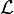$\mathcal{L}$ is often used as a variable for formal languages in logic.

Unlike natural languages, the symbols and formulas in formal languages are syntactically and semantically related to one another in a precise way. As a result, formal languages are completely (or almost completely) void of ambiguity.

## Examples

Some examples of formal languages include:

• The set of all words over${a, b}\,$
• The set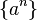$\left \{ a^{n}\right\}$, where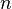$n\,$ is a natural number and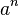$a^n\,$ means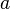$a\,$ repeated$n$ times
• Finite languages, such as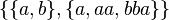$\{\{a,b\},\{a, aa, bba\}\}\,$
• The set of syntactically correct programs in a given programming language
• The set of inputs upon which a certain Turing machine halts

## Specification

A formal language can be specified in a great variety of ways, such as:

## Related pages

, chapter 6 Algebra of formalized languages.Formal language Facts for Kids. Kiddle Encyclopedia.